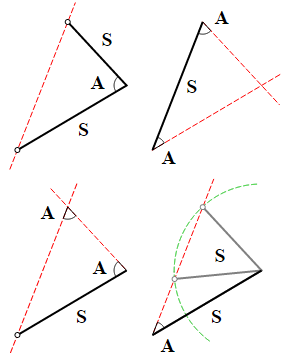# Triangle Area Sum 2

Geometry Level 5

Find all possible areas of a triangle with $2$ sides of lengths $11$ and $12$ and one $60^{\circ}$ angle.

If the sum of the areas of the $3$ smallest triangles is $a\sqrt{b},$ where $a$ and $b$ are integers and $b$ is square-free, enter your answer as $a+b$.×